# Divisor

Given two integers d and a, where d is nonzero, d is said to divide a, or d is said to be a divisor of a, if and only if there is an integer k such that dk = a. For example, 3 divides 6 because 3 · 2 = 6. Here 3 and 6 play the roles of d and a, while 2 plays the role of k. Though any number divides itself (as does its negative), it is said not to be a proper divisor. The number 0 is not considered to be a divisor of any integer.

More examples:

6 is a divisor of 24 since$6 \cdot 4 = 24$. (We stress that "6 divides 24" and "6 is a divisor of 24" mean the same thing.)
5 divides 0 because$5 \cdot 0 = 0$. In fact, every integer except zero divides zero.
7 is a divisor of 49 since$7 \cdot 7 = 49$.
7 divides 7 since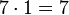$7 \cdot 1 = 7$.
1 divides 5 because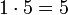$1 \cdot 5 = 5$.
−3 divides 9 because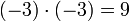$(-3) \cdot (-3) = 9$
−4 divides −16 because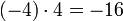$(-4) \cdot 4 = -16$
2 does not divide 9 because there is no integer k such that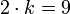$2 \cdot k = 9$. Since 2 is not a divisor of 9, 9 is said to be an odd integer, or simply an odd number.
• Given that d is non zero, the number k such that dk = a is unique and is called the exact quotient of a by d, denoted a/d.
• 0 can never be a divisor of any number. It is true that 0 · k = 0 for any k, however, the quotient 0/0 is not defined, as any k would work. This is the reason 0 is excluded from being considered a divisor.

## Notation

If d is a divisor of a (we also say that d divides a), this fact may be expressed by writing d | a. Similarly, if d does not divide a, we write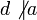$d \not| a$. For example, 4 | 12 but$8 \not| 12$.

## Related concepts

If d is a divisor of a (d | a), we say a is a multiple of d. For example, since 4 | 12, 12 is a multiple of 4. If both d1 and d2 are divisors of a, we say a is a common multiple of d1 and d2. Ignoring the sign (i.e., only considering nonnegative integers), there is a unique greatest common divisor of any two integers a and b written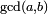$\scriptstyle\operatorname{gcd}(a, b)$ or, more commonly, (a,b). The greatest common divisor of 12 and 8 is 4, the greatest common divisor of 15 and 16 is 1. Two numbers with a greatest common divisor of 1 are said to be relatively prime. Complementary to the notion of greatest common divisor is least common multiple. The least common multiple of a and b is the smallest (positive) integer m such that a | m and b | m. Thus, the least common multiple of 12 and 9 is 36 (written [12,9] = 36).

## Abstract divisors

In higher mathematics, the notion of divisor has been abstracted from the integers to the context of general commutative rings. In this setting, they might be termed abstract divisors.Some content on this page may previously have appeared on Citizendium.